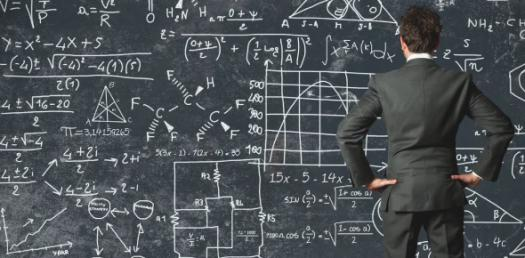# Place Value: 1st Grade Math Quiz

Approved & Edited by ProProfs Editorial Team
At ProProfs Quizzes, our dedicated in-house team of experts takes pride in their work. With a sharp eye for detail, they meticulously review each quiz. This ensures that every quiz, taken by over 100 million users, meets our standards of accuracy, clarity, and engagement.
| Written by Mkekb
M
Mkekb
Community Contributor
Quizzes Created: 1 | Total Attempts: 1,455
Questions: 10 | Attempts: 1,459SettingsBelow is a place value 1st Grade Math Quiz. In 1st grade, we get to learn how to describe and write numbers whether it is in ones, tens, hundreds, thousands. Do you think that you can easily identify the place value of a number in a sequence? Well, take up this quiz and find out for sure. All the best!

• 1.

### In the number 1,233, which number is listed in the tens place value?

Explanation
In the number 1,233, the number listed in the tens place value is 3. The tens place is the second digit from the right, and in this case, it is occupied by the number 3.

Rate this question:

• 2.

### In the number 4,002 which number is in the hunreds place value?

Explanation
The number 4,002 has 0 in the hundreds place value. The hundreds place is the third digit from the right in a four-digit number.

Rate this question:

• 3.

### In the number 12,309, which number is in the ones place value?

Explanation
In the number 12,309, the ones place value is represented by the digit 9. This means that 9 is the digit that occupies the rightmost position in the number and has a value of 9 units.

Rate this question:

• 4.

### In the number 18,436, which number is the tens place value?

Explanation
The tens place value in the number 18,436 is 3. The tens place value is the second digit from the right in a whole number. In this case, the digit 3 is in the tens place, indicating that there are 3 groups of ten in the number 18,436.

Rate this question:

• 5.

### in the number 21, 234which number is in the thousands place value?

Explanation
The number 1 is in the thousands place value because it is the first digit to the left of the decimal point. In the given number, 21,234, the thousands place value is represented by the digit 1.

Rate this question:

• 6.

### In the number 49, 293, which number is in the  Ten Thousand place value?

Explanation
The number 49,293 is a five-digit number. The Ten Thousand place value is the fourth digit from the left. In this case, the digit in the Ten Thousand place value is 4.

Rate this question:

• 7.

### In the number 23, 677, which number is in the tens place  value?

Explanation
In the number 23,677, the number 7 is in the tens place value. The tens place is the second digit from the right in a number and represents a value ten times greater than the ones place. In this case, the number 7 is in the tens place, indicating that it represents 70.

Rate this question:

• 8.

### Please wirte the number 4, 235 in expanded notation. Example: 123 would be 1 X100+ 2X10+ 3X1

Explanation
The given number, 4,235, is written in expanded notation as 4 x 1000 = 2 x 100 + 3 x 10 + 5 x 1. In expanded notation, each digit is multiplied by its corresponding place value and then added together to get the total value of the number.

Rate this question:

• 9.

### Please riight the number 402 in expaned notation

Explanation
The given answer correctly represents the number 402 in expanded notation. It shows that 402 can be written as 4 multiplied by 100, 0 multiplied by 10, and 2 multiplied by 1. This notation breaks down the number into its place values, making it easier to understand and work with.

Rate this question:

• 10.

### Please wite the number 9, 835 in expaned notation.

Explanation
The given number is 9,835. In expanded notation, we break down the number into its place values. The first digit, 9, is in the thousands place, so it is multiplied by 1000. The second digit, 8, is in the hundreds place, so it is multiplied by 100. The third digit, 3, is in the tens place, so it is multiplied by 10. The last digit, 5, is in the ones place, so it is multiplied by 1. Adding all these products together, we get 9x1000 + 8x100 + 3x10 + 5x1, which equals 9,000 + 800 + 30 + 5 = 9,835.

Rate this question:

Related TopicsBack to top
×

Wait!
Here's an interesting quiz for you.